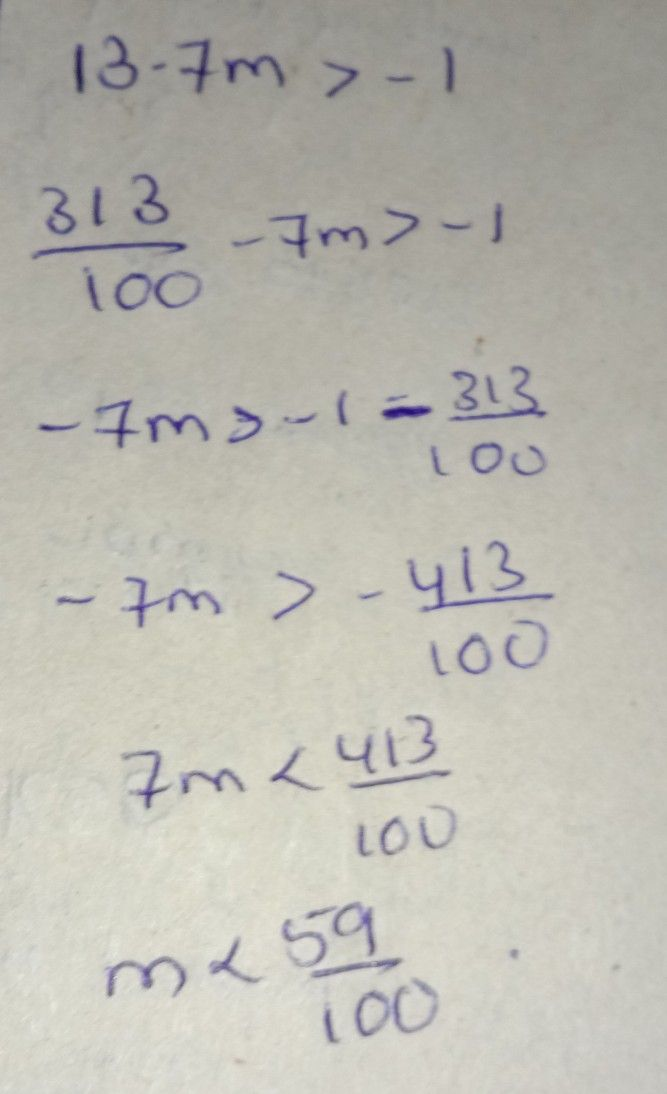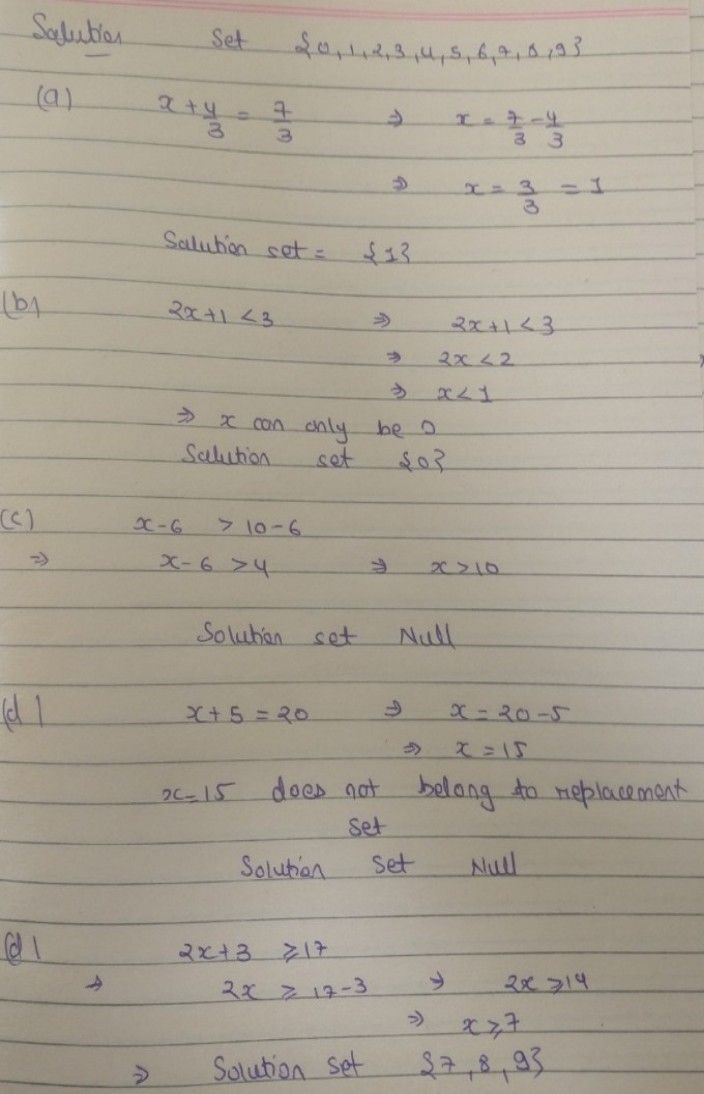Symbol
ProblemActivity $1.1:$ What Satisfies Me? $Diecti0ns$ Find the $solation/s$ of the following mathematical sentences. $csxx$ these mathematical sentences. $1.b+3<10$ $4r^{2}+6r-7=0$ $2.4c-5\geq 19$ $5.2m^{2}=50$ $3$ $13-7m>-1$ $6,$ $n^{2}-7n=-20$ $C$ What's New
7th-9th grade
Other
Search count: 107
SolutionQanda teacher - ruchiraStudent
is that the answer ma'am?